# 14 Partial Derivatives Copyright Cengage Learning All rights

• Slides: 21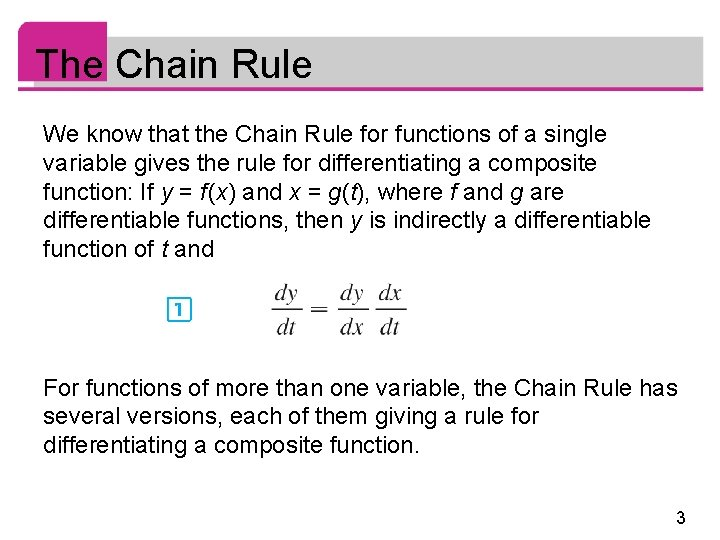The Chain Rule We know that the Chain Rule for functions of a single variable gives the rule for differentiating a composite function: If y = f (x) and x = g(t), where f and g are differentiable functions, then y is indirectly a differentiable function of t and For functions of more than one variable, the Chain Rule has several versions, each of them giving a rule for differentiating a composite function. 3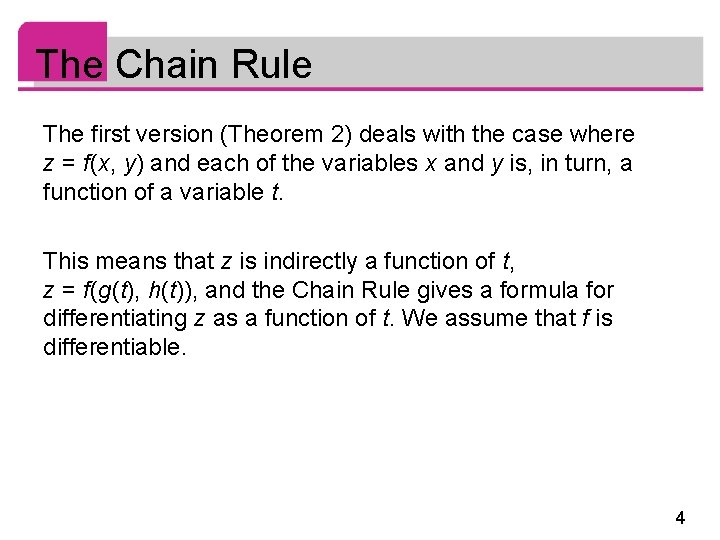The Chain Rule The first version (Theorem 2) deals with the case where z = f (x, y) and each of the variables x and y is, in turn, a function of a variable t. This means that z is indirectly a function of t, z = f (g(t), h(t)), and the Chain Rule gives a formula for differentiating z as a function of t. We assume that f is differentiable. 4The Chain Rule We know that this is the case when fx and fy are continuous. Since we often write ∂z/∂x in place of ∂ f /∂x, we can rewrite the Chain Rule in the form 5Example 1 If z = x 2 y + 3 xy 4, where x = sin 2 t and y = cos t, find dz/dt when t = 0. Solution: The Chain Rule gives It’s not necessary to substitute the expressions for x and y in terms of t. 6Example 1 – Solution cont’d We simply observe that when t = 0, we have x = sin 0 = 0 and y = cos 0 = 1. Therefore 7The Chain Rule We now consider the situation where z = f (x, y) but each of x and y is a function of two variables s and t: x = g(s, t), y = h(s, t). Then z is indirectly a function of s and t and we wish to find ∂z /∂s and ∂z /∂t. We know that in computing ∂z /∂t we hold s fixed and compute the ordinary derivative of z with respect to t. Therefore we can apply Theorem 2 to obtain 8The Chain Rule A similar argument holds for ∂z /∂s and so we have proved the following version of the Chain Rule. Case 2 of the Chain Rule contains three types of variables: s and t are independent variables, x and y are called intermediate variables, and z is the dependent variable. 9The Chain Rule Notice that Theorem 3 has one term for each intermediate variable and each of these terms resembles the one-dimensional Chain Rule in Equation 1. To remember the Chain Rule, it’s helpful to draw the tree diagram in Figure 2 10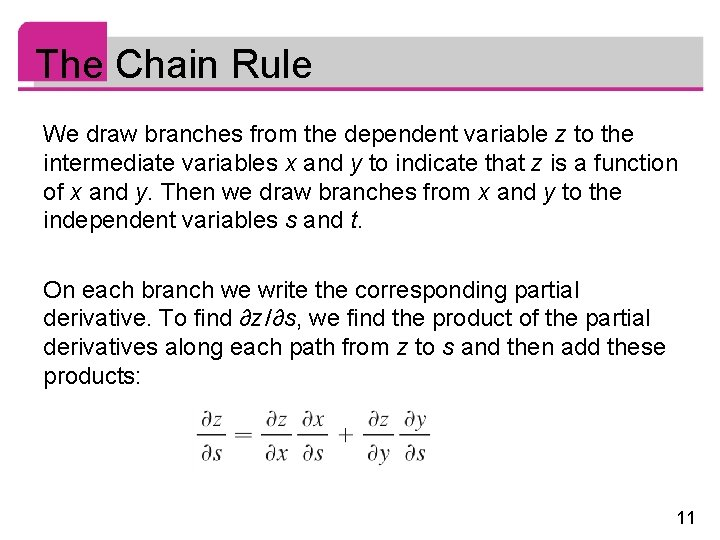The Chain Rule We draw branches from the dependent variable z to the intermediate variables x and y to indicate that z is a function of x and y. Then we draw branches from x and y to the independent variables s and t. On each branch we write the corresponding partial derivative. To find ∂z /∂s, we find the product of the partial derivatives along each path from z to s and then add these products: 11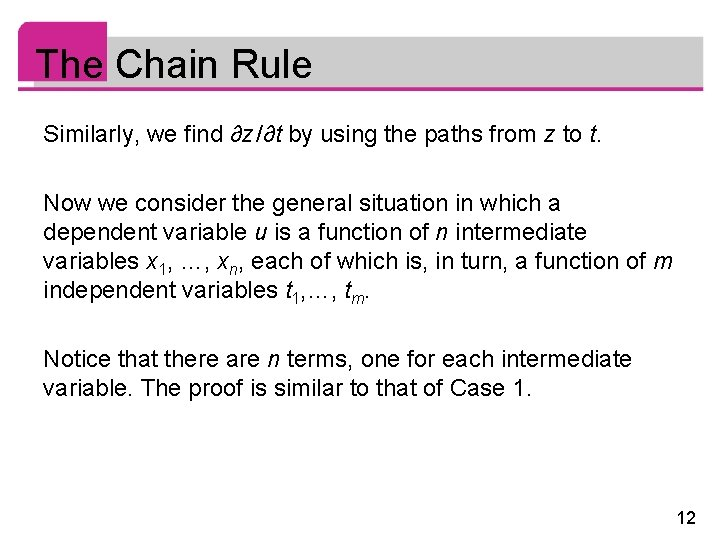The Chain Rule Similarly, we find ∂z /∂t by using the paths from z to t. Now we consider the general situation in which a dependent variable u is a function of n intermediate variables x 1, …, xn, each of which is, in turn, a function of m independent variables t 1, …, tm. Notice that there are n terms, one for each intermediate variable. The proof is similar to that of Case 1. 12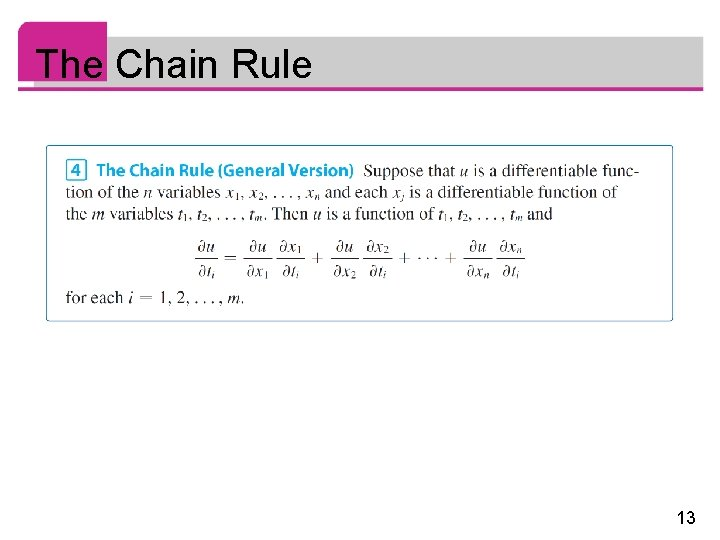The Chain Rule 13Implicit Differentiation 14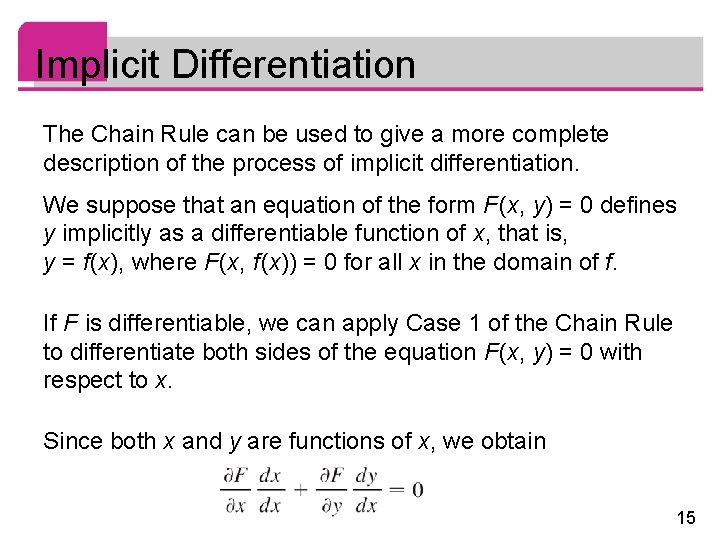Implicit Differentiation The Chain Rule can be used to give a more complete description of the process of implicit differentiation. We suppose that an equation of the form F (x, y) = 0 defines y implicitly as a differentiable function of x, that is, y = f (x), where F (x, f (x)) = 0 for all x in the domain of f. If F is differentiable, we can apply Case 1 of the Chain Rule to differentiate both sides of the equation F (x, y) = 0 with respect to x. Since both x and y are functions of x, we obtain 15Implicit Differentiation But dx /dx = 1, so if ∂F /∂x ≠ 0 we solve for dy /dx and obtain To derive this equation we assumed that F (x, y) = 0 defines y implicitly as a function of x. 16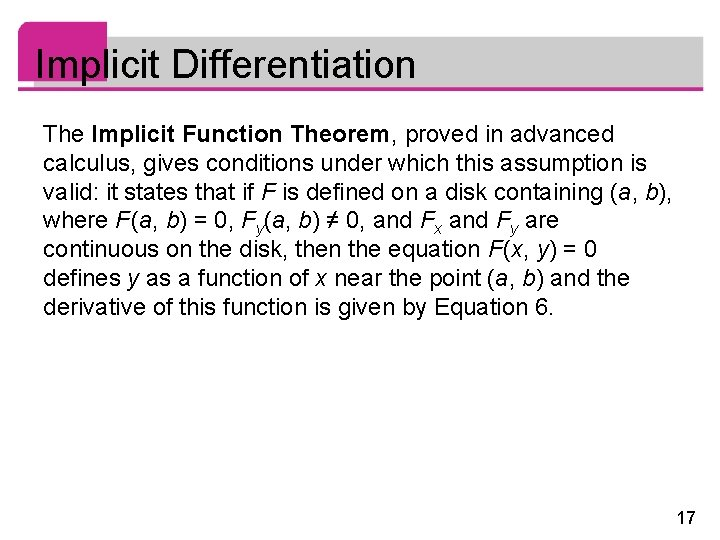Implicit Differentiation The Implicit Function Theorem, proved in advanced calculus, gives conditions under which this assumption is valid: it states that if F is defined on a disk containing (a, b), where F (a, b) = 0, Fy(a, b) ≠ 0, and Fx and Fy are continuous on the disk, then the equation F (x, y) = 0 defines y as a function of x near the point (a, b) and the derivative of this function is given by Equation 6. 17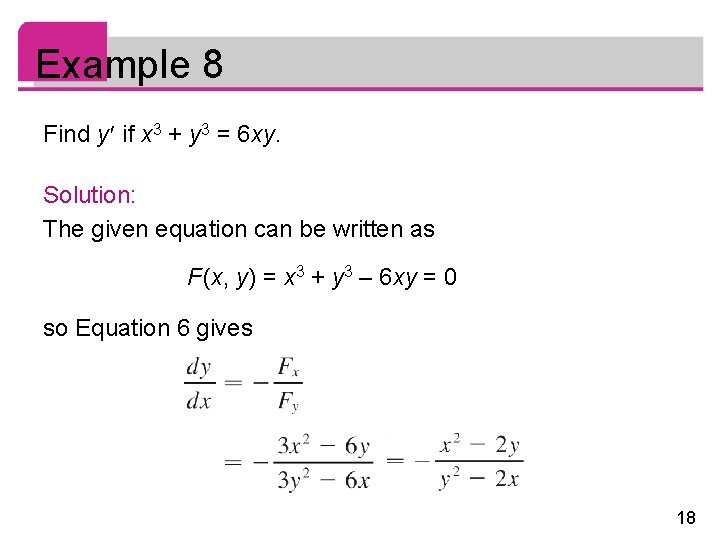Example 8 Find y if x 3 + y 3 = 6 xy. Solution: The given equation can be written as F (x, y) = x 3 + y 3 – 6 xy = 0 so Equation 6 gives 18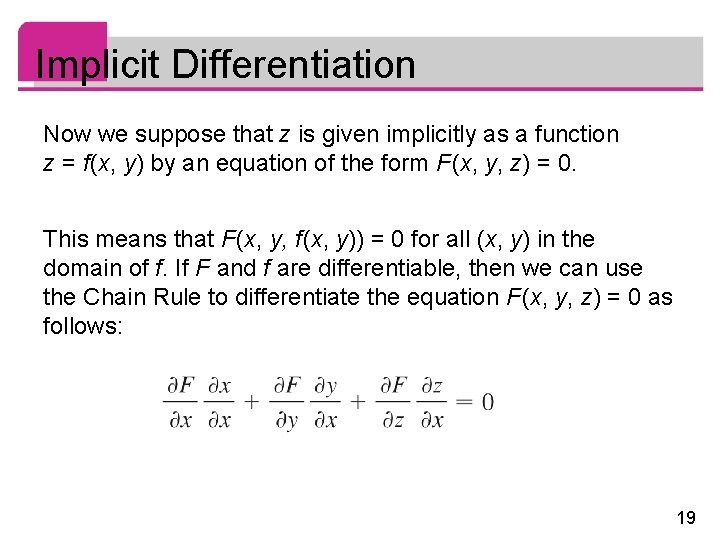Implicit Differentiation Now we suppose that z is given implicitly as a function z = f (x, y) by an equation of the form F (x, y, z) = 0. This means that F (x, y, f (x, y)) = 0 for all (x, y) in the domain of f. If F and f are differentiable, then we can use the Chain Rule to differentiate the equation F (x, y, z) = 0 as follows: 19Implicit Differentiation But and so this equation becomes If ∂F /∂z ≠ 0, we solve for ∂z /∂x and obtain the first formula in Equations 7. The formula for ∂z /∂y is obtained in a similar manner. 20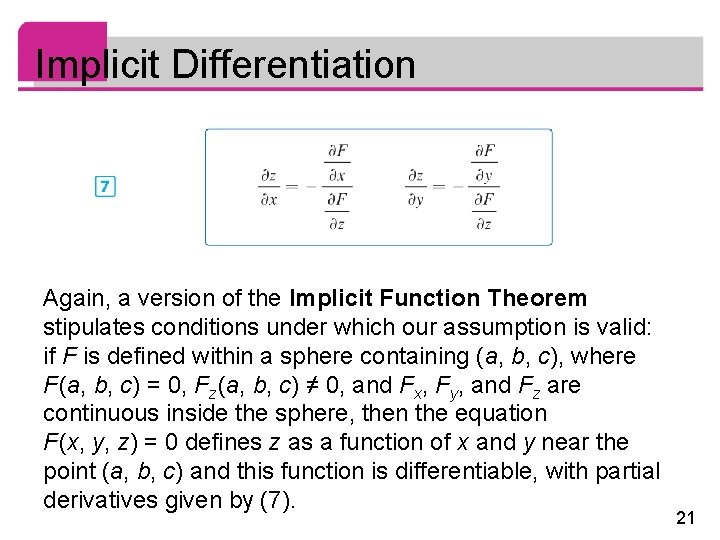Implicit Differentiation Again, a version of the Implicit Function Theorem stipulates conditions under which our assumption is valid: if F is defined within a sphere containing (a, b, c), where F (a, b, c) = 0, Fz (a, b, c) ≠ 0, and Fx, Fy, and Fz are continuous inside the sphere, then the equation F (x, y, z) = 0 defines z as a function of x and y near the point (a, b, c) and this function is differentiable, with partial derivatives given by (7). 21